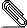# Re: [HACKERS] Path question

```On Sep 2, 2010, at 1:20 AM, Robert Haas wrote:

> On Sep 1, 2010, at 10:21 AM, Greg Stark <gsst...@mit.edu> wrote:
>> For what it's worth I disagree with Tom. I think this is a situation
>> where we need *both* types of solution. Ideally we will be able to use
>> a plain Append node for cases where we know the relative ordering of
>> the data in different partitions, but there will always be cases where
>> the structured partition data doesn't actually match up with the
>> ordering requested and we'll need to fall back to a merge-append node.
>
> I agree. Explicit partitioning may open up some additional optimization
> possibilities in certain cases, but Merge Append is more general and
> extremely valuable in its own right.
>
> ...Robert
> --
> Sent via pgsql-hackers mailing list (pgsql-hackers@postgresql.org)
> To make changes to your subscription:
> http://www.postgresql.org/mailpref/pgsql-hackers```
```

we have revised greg's wonderful work and ported the entire thing to head.
it solves the problem of merge_append. i did some testing earlier on today and
it seems most important cases are working nicely.

here are some test cases:

test=# \d t_data
Table "public.t_data"
Column |  Type   | Modifiers
--------+---------+-----------
id     | integer |
tstamp | date    |

test=# \d t_data_1
Table "public.t_data_1"
Column |  Type   | Modifiers
--------+---------+-----------
id     | integer |
tstamp | date    |
Indexes:
"idx_1" btree (id)
Check constraints:
"t_data_1_id_check" CHECK (id >= 1 AND id <= 10000)
Inherits: t_data

test=# \d t_data_2
Table "public.t_data_2"
Column |  Type   | Modifiers
--------+---------+-----------
id     | integer |
tstamp | date    |
Indexes:
"idx_2" btree (id)
Check constraints:
"t_data_2_id_check" CHECK (id >= 10001 AND id <= 20000)
Inherits: t_data

test=# \d t_data_3
Table "public.t_data_3"
Column |  Type   | Modifiers
--------+---------+-----------
id     | integer |
tstamp | date    |
Indexes:
"idx_3" btree (id)
Check constraints:
"t_data_3_id_check" CHECK (id >= 20001 AND id <= 30000)
Inherits: t_data

simple windowing ...

test=# explain select *, max(id) OVER ( ORDER BY id) from t_data ;
QUERY PLAN

-----------------------------------------------------------------------------------------------------
WindowAgg  (cost=149.99..2154.43 rows=32140 width=8)
->  Result  (cost=149.99..1672.33 rows=32140 width=8)
->  Append  (cost=149.99..1672.33 rows=32140 width=8)
->  Sort  (cost=149.78..155.13 rows=2140 width=8)
Sort Key: public.t_data.id
->  Seq Scan on t_data  (cost=0.00..31.40 rows=2140
width=8)
->  Index Scan using idx_1 on t_data_1 t_data
(cost=0.00..318.25 rows=10000 width=8)
->  Index Scan using idx_2 on t_data_2 t_data
(cost=0.00..318.25 rows=10000 width=8)
->  Index Scan using idx_3 on t_data_3 t_data
(cost=0.00..318.25 rows=10000 width=8)
(9 rows)

it does a nice index scan; merges the stuff and puts it up into the high level
doing the windowing.

test=# select *, max(id) OVER ( ORDER BY id) from t_data LIMIT 10;
id |   tstamp   | max
----+------------+-----
1 | 2010-01-01 |   1
2 | 2010-01-01 |   2
3 | 2010-01-01 |   3
4 | 2010-01-01 |   4
5 | 2010-01-01 |   5
6 | 2010-01-01 |   6
7 | 2010-01-01 |   7
8 | 2010-01-01 |   8
9 | 2010-01-01 |   9
10 | 2010-01-01 |  10
(10 rows)

the cost model does what it should as well:

test=# explain select *, max(id) OVER ( ORDER BY id) from t_data ;
QUERY PLAN

---------------------------------------------------------------------------------------------
WindowAgg  (cost=2872.41..3434.86 rows=32140 width=8)
->  Sort  (cost=2872.41..2952.76 rows=32140 width=8)
Sort Key: public.t_data.id
->  Result  (cost=0.00..466.40 rows=32140 width=8)
->  Append  (cost=0.00..466.40 rows=32140 width=8)
->  Seq Scan on t_data  (cost=0.00..31.40 rows=2140
width=8)
->  Seq Scan on t_data_1 t_data  (cost=0.00..145.00
rows=10000 width=8)
->  Seq Scan on t_data_2 t_data  (cost=0.00..145.00
rows=10000 width=8)
->  Seq Scan on t_data_3 t_data  (cost=0.00..145.00
rows=10000 width=8)
(9 rows)

it has proven to be really valuable in my first tests.
maybe this is helpful for some people out there.

many thanks,

hans

```merge-append-91-v1.diff
Description: Binary data

```
--
Cybertec Schönig & Schönig GmbH
Gröhrmühlgasse 26
```--